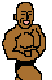## R Aqr: Analysis of the AFOEV database

(posted by. I. Andronov, vsnet 858)

\documentstyle[11pt]{article}
\textwidth 170mm
\textheight 230mm
\oddsidemargin -3mm
\topmargin -16mm
\begin{document}
\begin{center}

{\Large\bf PULSATIONS OF THE MIRA--TYPE COMPONENT\\ IN THE SYMBIOTIC BINARY R AQR}

\footnote{IAU Symposium 180 "Planetary Nebulae" Poster III-1}\\[1mm] L.L. Chinarova$^1,$ I.L. Andronov$^1,$ E. Schweitzer$^2$\\[1mm] $^1$Department of Astronomy, Odessa State University, T.G.Shevchenko Park, Odessa 270014 Ukraine\\ $^2$AFOEV, Strasbourg, 11, rue de l'Universit\'e, 67000 Strasbourg, France\\[2mm] \end{center}

{\bf Abstract}. Time series analysis of the brightness variations of R Aqr is based on 1521 visual observations from the AFOEV database. The statistically significant number of harmonics for the mean phase curve is $m=5.$ The cycle--to--cycle variations of the phase curve are present. The most stable in phase is the maximum, for which no secular period variations detected, but a secondary cycle of $P_2=3955\pm50^d$ and amplitude $(0.032\pm0.005)P$ may be suggested.\\[2mm]

R Aqr is a prototype of the subclass of symbiotic binaries the light variations which are characteristic for a long-periodic variable. It exhibits characteristics of many types of astrophysical objects in the same system. This work continues our study of symbiotic variables with Mira--type components. Previous results were published by Chinarova et al. (1994), and Chinarova (1995).

The observations were made by the members of the AFOEV in 1935--1996 (JD 2428088-50096). The AFOEV database was described by Schweitzer (1993). From all data we deleted not sure values and estimates "fainter than", thus 1521 data points remained. The most numerous data were obtained by the following observers: L.Bichon (62), M.Duruy (150), R.Fidrich (63), A.Mizser (94), S.Papp (46), M.Petit (46), P.Schmidt (57), M.Silhol (53), B.Thouet (83), P.Vedrenne (130). In the brackets after the name one may find the number of his observations. We thank these amateur astronomers for their intensive studies.

The data set was analysed by using the the computer code FOUR-N (Andronov, 1994). The number of statistically significant harmonics $m=5$ was determined by using the Fischer's test.

The mean epoch for maximum $(6.78\pm0.03^m)$ is JD $2444733.76\pm0.93^d,$ minimum $(11.15\pm0.09^m)$ JD $2444575.1\pm4.0^d.$ The asymmetry $A=0.409\pm0.011$ coincides with the value $A=0.42$ listed in the GCVS, despite the best fit period value $P=387.509\pm0.029^d$ significantly differs from that 386.96 listed in GCVS. One may note an apparent hump at the ascending branch. It is not seen at all individual cycles, but is statistically significant at the mean phase curve.

The extremal slopes of the fit occur at the phases computed in respect to the maximum are $\phi=-0.098$ (ascending branch, $dt/dm=15.4\pm0.5$ days/mag) and $\phi=0.217$ (descending branch, $dt/dm=30.6\pm2.5$ days/mag. The ratio of the slope to that expected for the sine with the same period and full amplitude is $B=1.84\pm0.06$ and $B=0.92\pm0.08$), respectively.

One may note the cycle--to--cycle changes of the phase curve shape. To fit the variations in the individual cycles we have used several fitting routines as briefly described by Andronov (1996): "running parabolae" (RP), "running sines" (RS), "asymptotic parabolae" (AP) and "linear fit" (LF) approximations.

The optimal value of the filter half-width $\Delta t$ for "RP" fit was found to be $\Delta t=57^d$ corresponding to the maximal "signal/noise" ratio S/N=17.55. The unbiased estimate of the r.m.s. deviation of the observations from the fit is $\sigma_0=0.24^m$ which is in a reasonable agreement with the accuracy of visual observations. The r.m.s. accuracy of the fit $0.08^m.$ However, the observational gaps are large, thus we have used additional methods to check the results.

The method of "running sines" was proposed by Chinarova et al. (1994) which was applied to the Mira-type component in the symbiotic binary UV Aur. However, the curve of R Aqr is asymmetric, thus one may suggest to extend the method of "running sines" to "running multiharmonic fit". The larger number of harmonics corresponds to better fit at the branches of the light curve well covered by the observations, but the uncertainty of the "interpolated" branches far from data is significantly larger and one may see apparent false high--amplitude variations.

The method of "asymptotic parabolae" was found to be the best for our type of gapped data. It is efficient for the curves with nearly linear ascending and descending branches, the transition between which may be fitted by a parabola.

Linear fits $t(m)$ were used to determine the times of the crossings of the fit and a constant value (set to $9^m$) and the corresponding slopes $dt/dm.$ Such additional moments are more numerous for R Aqr (20 for descending branch, 22 for ascending branch as compared with 16 maxima and 7 minima).

The mean characteristics of the parameters obtained by means of "LF" are: standard error of the determination of time $1.46^d=0.0038P,$ standard deviation of the brightness from the linear fit $0.235^m$ in exellent agreement with the value $0.239^m$ estimated from the "RP" fit with optimal value of the filter half-width $\Delta t.$ The mean slopes are $t_i=16.8\pm1.5^d$ and $t_d=39.6\pm1.1^d$ for incline and decline, respectively. The value of $t_i$ is close to that obtained from the 5--harmonic fit $(15.4\pm0.5),$ but $t_d$ differs significantly from $30.6\pm2.5.$

The range of variations is $5.7-8.0^m$ (brightness at maximum), $10.6-12.3^m$ (brightness at minimum), $7.1-34.1$ days/mag (slope of the ascending branch at $m=9^m$), $25.7-44.6$ days/mag (slope of the descending branch at $m=9^m$). The corresponding full amplitudes of the phase variations are 0.069, 0.136, 0.120, 0.256, respectively. The most stable in phase is the maximum. No secular period variations was detected, but one may suggest a secondary cycle (third body?) of $P_2=3955\pm50^d$ and amplitude $(0.032\pm0.005)P$ for the phases of maxima.

One may introduce the parameter $A_l=t_i/(t_i+t_d)$ equal to the asymmetry, if the light curve is composed of the linear incline and decline with zero-length transitions. For R Aqr its mean value is equal to $0.298\pm0.019.$ For the 5--harmonic fit $A_l=0.335\pm0.020,$ i.e. the same as the "LF" value within error estimates.\\[2mm]

{\bf References}\\[2mm]
Andronov, I.~L., 1994, Odessa Astron. Publ., 7, 49.\\
Andronov, I.L., 1996, IAU Symp.180 "Planetary Nebulae",
Poster III-1 (in press).\\
Chinarova L.L., 1995, Astron. Astrophys. Transact., 9, 103.\\
Chinarova L.L., Andronov I.L., Schweitzer E., 1994,
Odessa Astron. Publ., 7, 103.\\
Schweitzer E., 1993, Bull. AFOEV, 64, 14.
\end{document}


vsnet-adm@kusastro.kyoto-u.ac.jp